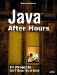Drawing Rectangles

If the rectangle flag is TRue, the user is drawing rectangles, and the code creates a Rectangle2D.Double rectangle to correspond to the ordered starting point, tempStart, and the figure's height and width. The Rectangle2D.Double constructor takes the same arguments as the Ellipse.Double constructor, so it works like this:

if(rectangle && width != 0 && height != 0){     Rectangle2D.Double rectangle =         new Rectangle2D.Double(tempStart.x, tempStart.y,         width, height); . . .

If the user wants a drop shadow, you should draw that first, which the code does like this:

if(shadow){         paint = gImage.getPaint();         composite = gImage.getComposite();         gImage.setPaint(Color.black);         gImage.setComposite(AlphaComposite.getInstance             (AlphaComposite.SRC_OVER, 0.3f));         Rectangle2D.Double rectangle2 =             new Rectangle2D.Double(tempStart.x + 9,             tempStart.y + 9, width, height);         if(solid || shade || transparent || texture){             gImage.fill(rectangle2);         }         else{             gImage.draw(rectangle2);         }         gImage.setPaint(paint);         gImage.setComposite(composite);     } . .

If the user wants a solid-filled rectangle, you can set the Graphics2D graphics context to use the current drawing color this way:

if(solid){         gImage.setPaint(color);     } . . .

If the shade flag is set, the user wants to fill the rectangle with a gradient fill. As before, the code will create a gradient ranging from the current drawing color to black:

if(shade){         gImage.setPaint(             new GradientPaint(             tempStart.x, tempStart.y, color,             tempEnd.x, tempEnd.y, Color.black));          } . . .

You can also create transparent rectangles by setting the graphics context's composite to make the drawing results transparent:

if(transparent){         gImage.setComposite(AlphaComposite.getInstance         (AlphaComposite.SRC_OVER,0.3f));     } . . .

And if the user wants a texture fill, you can set that up this way:

if(texture){         Rectangle2D.Double anchor =             new Rectangle2D.Double(0,0,             tileImage.getWidth(), tileImage.getHeight());         TexturePaint texturePaint =             new TexturePaint(tileImage,anchor);         gImage.setPaint(texturePaint);     } . . .

The final step is to actually draw the rectangle and restore the composite if need be:

if(solid || shade || texture || transparent){         gImage.fill(rectangle);     }     else{         gImage.draw(rectangle);     }     if(transparent){         gImage.setComposite(composite);     } }

You can see what a variety of rectangles looks like, with some graphics effects added, in Figure 4.4.

Figure 4.4. Various graphics effects with rectangles.Java After Hours: 10 Projects Youll Never Do at Work
ISBN: 0672327473
EAN: 2147483647
Year: 2006
Pages: 128

Similar book on Amazon